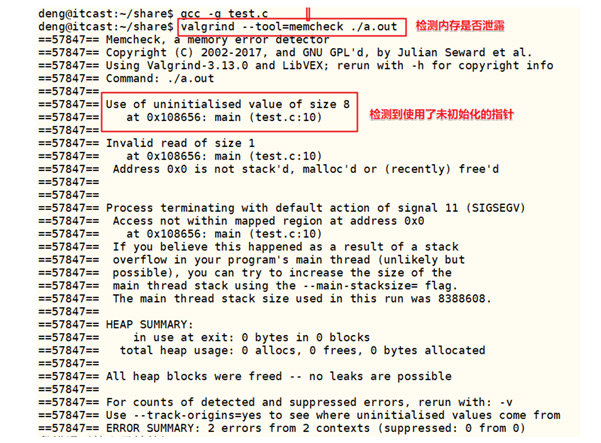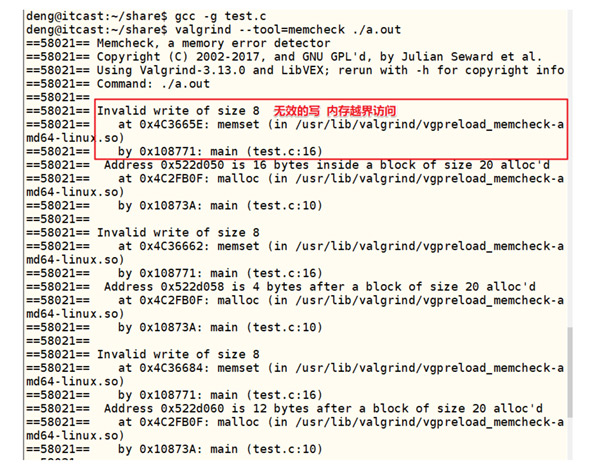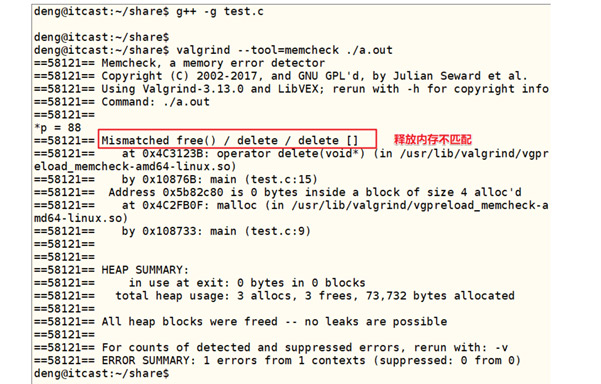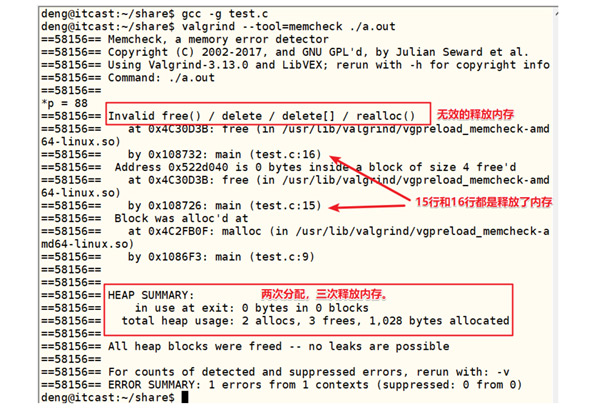# Linux下C和C++程序中内存泄露检测

01. 前言

C/C++运行高效，不管是操作系统内核还是对性有要求的程序（比如游戏引擎）都要求使用C/C++来编写，其实C/C++强大的一点在于能够使用指针自由地控制内存的使用，适时的申请内存和释放内存，从而做到其他编程语言做不到的高效地运行。但是内存管理是一把双刃剑，用好了削铁如泥，用不好自断一臂。在申请堆上内存使用完之后中如果做不到适时有效的释放，那么就会造成内存泄露，久而久之程序就会将系统内存耗尽，导致系统运行出问题。就如同你每天跑去图书馆借一打书籍而不还，直到图书馆倒闭为止。

C语言中申请内存和释放内存的方法是使用 malloc和free。

C++中能兼容C，所以也能使用malloc和free，面向对象的情况下使用的则是new和delete，能够自动执行构造函数和析构函数。

02. valgrind安装

debian/ubuntu下安装方法：

deng@itcast:~\$ sudo apt install valgrind
deng@itcast:~\$ sudo yum install valgrind

redhat/centos下安装方法：

03. 使用未初始化的内存

#include <stdio.h>
#include <string.h>
#include <stdlib.h>

int main(void)
{
char ch;
char *p;

ch = *p;

printf("ch = %c\n", ch);

return 0;
}

valgrind检测出到我们的程序使用了未初始化的变量。04. 使用野指针

p所指向的内存被释放了，p变成了野指针，但是我们却继续使用这片内存。

#include <stdio.h>
#include <string.h>
#include <stdlib.h>

int main(void)
{
int *p = NULL;

p = malloc(sizeof(int));
if (NULL == p)
{
printf("malloc failed...\n");
return 1;
}
memset(p, 0, sizeof(int));

*p = 88;

printf("*p = %d\n", *p);

//释放内存
free(p);

printf("*p = %d\n", *p);

return 0;
}

valgrind检测到我们使用了已经free的内存，并给出这片内存是哪里分配和哪里释放的。05. 动态内存越界访问

#include <stdio.h>
#include <string.h>
#include <stdlib.h>

int main(void)
{
int i = 0;
int *p = NULL;

p = malloc(5 * sizeof(int));
if (NULL == p)
{
printf("malloc failed...\n");
return 1;
}
memset(p, 0, 10 * sizeof(int));

for (int i = 0; i <= 5; i++)
{
p[i] = i + 1;
}

for (int i = 0; i <= 5; i++)
{
printf("p[%d]: %d\n", i, p[i]);
}

return 0;
}

valgrind检测出越界信息如下。valgrind不检查非动态分配数组的使用情况。

06. 分配空间后没有释放

#include <stdio.h>
#include <string.h>
#include <stdlib.h>

int main(void)
{
int *p = NULL;

p = malloc(sizeof(int));

*p = 88;

printf("*p = %d\n", *p);

return 0;
}

valgrind的记录显示上面的程序用了1次malloc，却调用了0次free。07. 不匹配使用delete或者free

#include <stdio.h>
#include <string.h>
#include <stdlib.h>

int main(void)
{
int *p = NULL;

p = (int *)malloc(sizeof(int));

*p = 88;

printf("*p = %d\n", *p);

delete p;

return 0;
}08. 两次释放同一块内存

#include <stdio.h>
#include <string.h>
#include <stdlib.h>

int main(void)
{
int *p = NULL;

p = (int *)malloc(sizeof(int));

*p = 88;

printf("*p = %d\n", *p);

free p;
free p;

return 0;
}09. 总结

· 未释放内存的使用

· 对释放后内存的读/写

· 对已分配内存块尾部的读/写

· 内存泄露

· 不匹配的使用malloc/new/new[] 和 free/delete/delete[]

· 重复释放内存

c语言入门教程下载

## 相关阅读